## Posts

Showing posts from 2010

### Geometrical ProofsIntroduction Intercept theorem There are 2 ways to apply the intercept theorem In the first scenario, you will be able to identify a pair of parallel lines from the question Upon identifying the pair of parallel lines, we conclude that the ratio of AD:DB is the same as the ratio of AE:EC In the second scenario, you identify that that the ratio of AD:DB is the same as the ratio of AE:EC Subsequently you conclude that the lines DE and BC are parallel Midpoint Theorem The Midpoint theorem is a specific case of the Intercept theorem when the ratio of the lengths is 1:1 There are 2 ways to apply the Midpoint Theorem The first way is to use Midpoint Theorem to show that 2 lines are parallel From the information given in the question, we will be able to identify that D is the midpoint of AB and E is the midpoint of AC Given that D and E are midpoints, we will be able to conclude that DE is parallel to BC The second way is to use Midpoint Theorem to show that E is a midpoint From th

### Integration techniques Part IIIIntegrating fractions Revision of anti chain rule "Increase power by 1 and divide by the new power" Recall the differentiation of ln (ax + b)

### Equation of Circles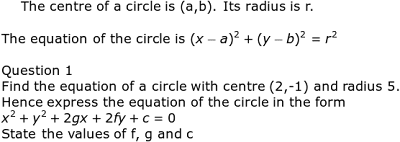Formula for Equation of Circle

### Completing the SquareCompleting the square is a technique required to find equations of circle Example 1

### Coordinate Geometry Part II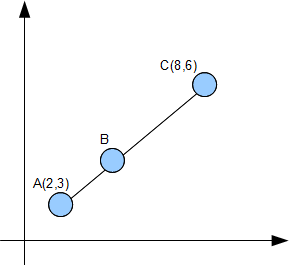Example 1 A typical coordinate geometry question from the O Levels Given that AC = 3AB, find the coordinates of point B. Remarks: A lot of students will try to use the distance formula to form simultaneous equations in x and y to find the coordinates of B. This is a very long and tedious method. A much simpler method is to use ratio and proportion to find the coordinates of B To find the x coordinate of B We can use the same method to find the y coordinate of point B Therefore the coordinates of point B is (4,4)

### Coordinate Geometry Part ITechnique 1: Finding the gradient of a line Parallel lines Parallel lines have the same gradient Perpendicular lines If a line has a gradient of 1/2 , the gradient of the line perpendicular to it is -2. -2 is the negative reciprocal of 1/2. In other words, we "flip" the fraction 1/2 to get 2 and attach a negative sign Technique 2: Finding equation of a straight line The general format of a straight line is y = mx + c where m is the gradient and, c is the y intercept Example 2 Find the equation of a line that contains the points (1,2) and (3,4). From the example above we know that gradient of the line is 1. Hence the equation of the line is: y = 1x + c To find the y intercept, c, we sub in one of the points I choose the point (1,2) and sub x =1, y = 2 2 = 1 + c Hence c = 1 The equation of the line is y = x + 1 Let us combine the techniques we have learnt so far to solve a typical O Level question Question 1 The figure above shows a trapezium where AB is parall

### Roots of Quadratic Equation Part II### Roots of a Quadratic Equation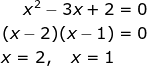What do we mean by the roots of a quadratic equation? Example 1 We say that 2 and 1 are roots of the equation. The equation has 2 real and distinct roots In graphical form, the roots of the equation are represented as the intersection of the curve with the x axis. Example 2 In this case there is only 1 solution. We say that the equation has only 2 real and equal roots Graphically it can be represented as Notice that there is only 1 intersection point between the curve and the x axis Example 3 Lastly we are going to look at an example where the curve does not intersect the x axis Consider the equation: The equation cannot be factorized. It cannot be solved using the quadratic formula. Graphically it is represented as It turns out that we can predict the number of roots that an equation has by looking at the discriminant Recall the quadratic formula

### Remainder theorem II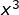Question 1 The coefficient of the term in a cubic polynomial f(x) is -1. The roots of f(x) are 1, 2 and k. f(x) when divided by x - 3 gives a remainder of 8. Find the value of k First we need to understand what is meant by roots of an equation

### Remainder theorem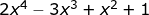Introduction How do we find the remainder when we divide by ? One way is to use long division From the long division, we get a remainder of 13 Note that: is known as the divisor is known as the quotient is known as the dividend In most cases we are only interested in the remainder, there is an easier way of obtaining the remainder without using long division The easier way is to use the Remainder Theorem Once again we want to find the remainder when is divided by How do we know what value to sub in ? If we are dividing by x - 2, we let x - 2 =0 and get x = 2. So we sub in 2 If we are dividing by x + 2, we let x + 2 =0 and get x = -2. So we sub in -2 If we are dividing by x - a, we let x - a =0 and get x = a. So we sub in a Question 1 Given that leaves a remainder of 6 when divided by and has a factor of , find the value of a and b. Next the question says that x + 2 is a factor Factor means that the remainder is zero Hence we can apply the remainder t

### Binomial Theorem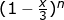Question If the first 3 terms of the expansion of are , find the value of x and n Solution Recall the binomial expansion formula Next we need to simplify Going back to the question, We can then form 2 equations involving n and x Equation 1 Equation 2

### Integration of Trig Functions Part II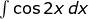Be careful of the negative signs when integrating sin and cos functions Question 1 a) Note the absence of the negative sign We divide by 2 as the differentiation of 2x is 2 b) Note the presence of the negative sign We divide by 2 as the differentiation of 2x is 2 Question 2 a) Note the presence of the negative sign b) Note the absence of the negative sign